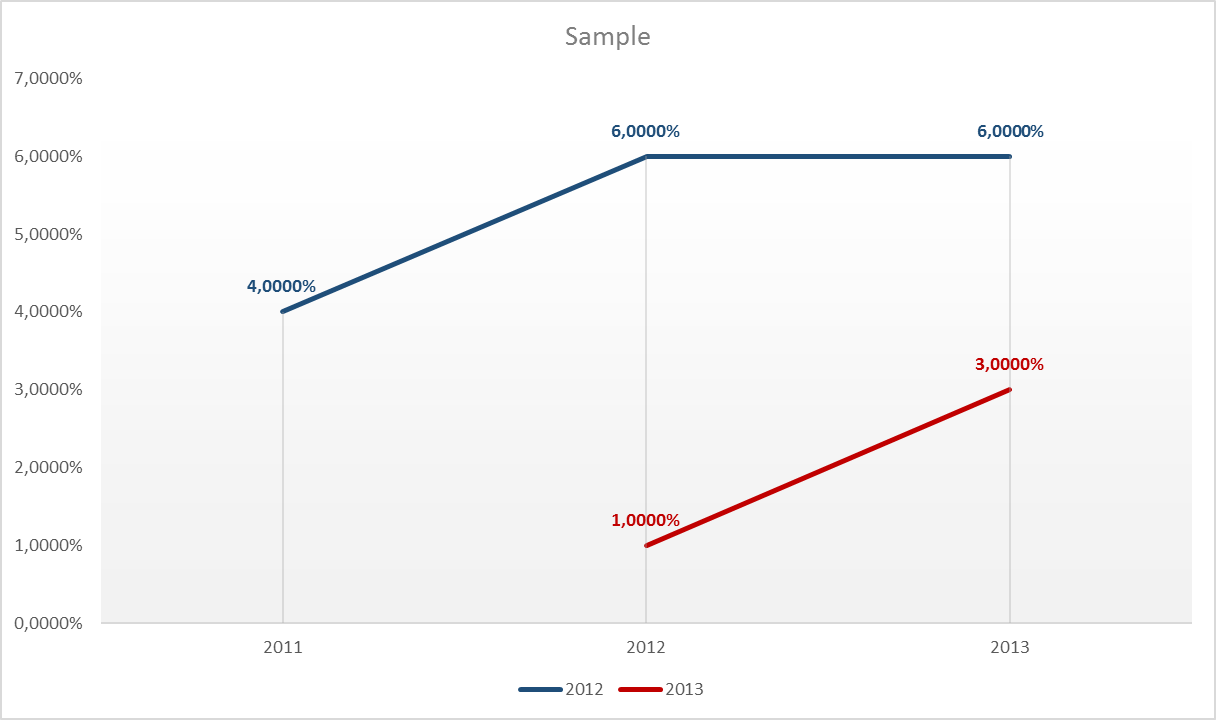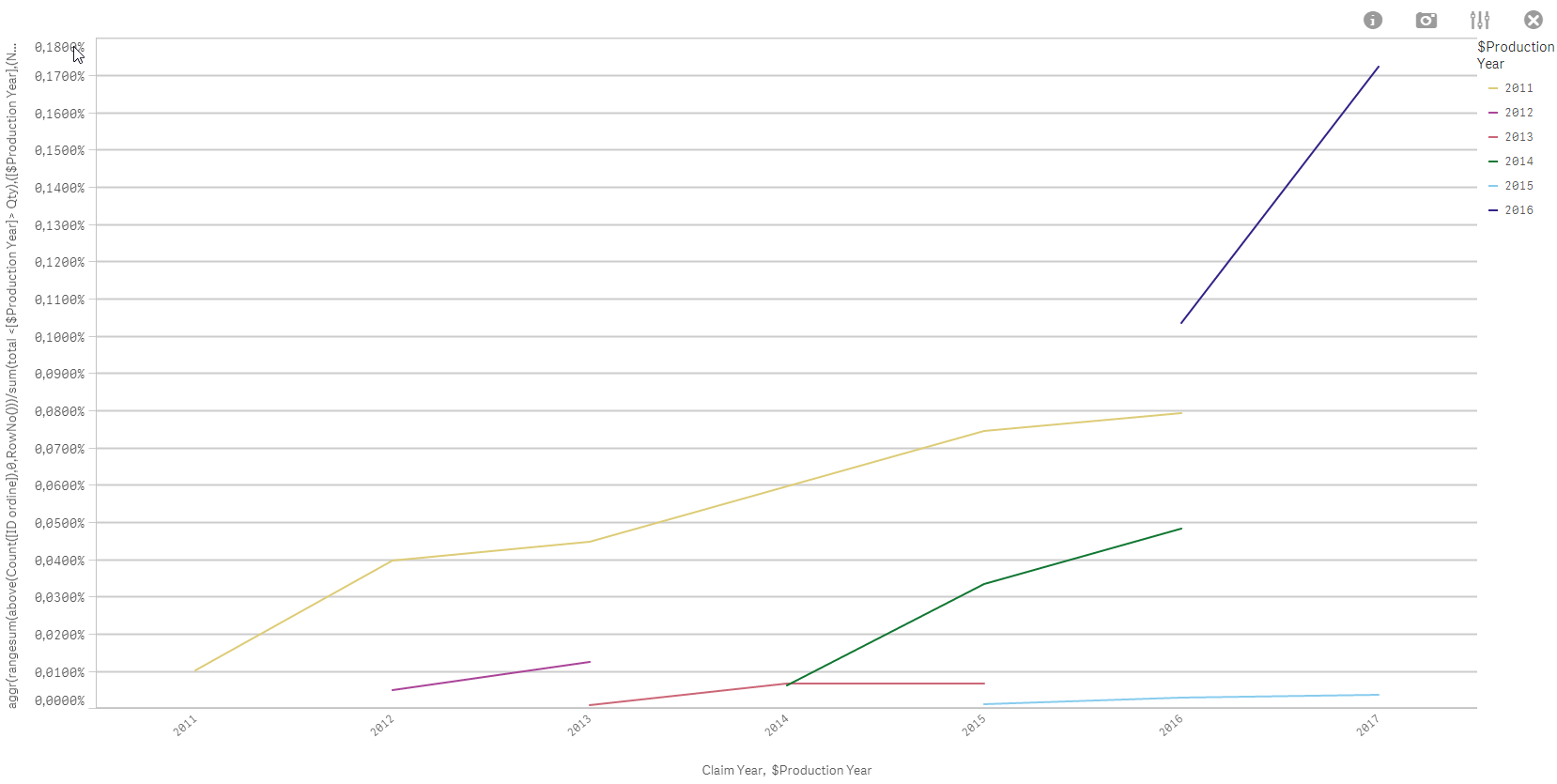# New to Qlik Sense

Discussion board where members can get started with Qlik Sense.

Highlighted
New Contributor

## Cumulative Percentage sum of a row obtained by a pivot table in Qlik Sense

Dear all,

I have a big issue and I think that what I have to do goes far beyond my competences.

I have loaded 2 tables in the script, there are other colums but I need only some of them to compute the calculation:

[Purchased]

ItemSupplierQuantityTurnoverYear

[Database]

idOrderItemSupplierMachine
Production year
Claim Year

What I need to do is to compute a cumulative sum of the percentage of defects of a single item ( count(idorder)/quantity), for a supplier;

more specifically:

in 2011 I produced and sold a machine;

In 2011 I received 4 claims (4 id.orders) of representing 2 different items [A and B] (for simplicity 2 claims per item); In 2012 i received 2 claims, regarding  in this case of the 2 different items considered before;

in 2012 i produced and sold another machine;

in 2012 i received 1 claim, in 2013 a recieved 2 claims.

I have in the table (purchased) the yearly quantity purchased of that specific item, for simplicity Production Year is the same of Purchased Year.

my index should be somthing like this:

Claim 2011claim 2012claim 2013Purchased
20114/100 = 4%2/100=2%0%100 (total sum of items)
2012
 1/100=1%
 2/100=2%
100 (total sum of items)

in a graph they would be represented like this:

y : cumulative sum

x : year of claimThis is what I have to do. In excel is simple but too much time consuming. I don't get something in order to do this in qlik sense.

Thank you in advance if anyone can help me

Tags (8)
1 Solution

Accepted Solutions
Valued Contributor III

## Re: Cumulative Percentage sum of a row obtained by a pivot table in Qlik Sense

I think I got it ... expression:

aggr(rangesum(above(Count([ID ordine]),0,RowNo()))/sum(total <[\$Production Year]> Qty),([\$Production Year],(NUMERIC, ASCENDING)),([Claim Year],(NUMERIC, ASCENDING)))12 RepliesPartner

## Re: Cumulative Percentage sum of a row obtained by a pivot table in Qlik Sense

If your expression is sum(kpi); to do the cumul :

rangesum(above(sum(kpi),0,rowno())

Hope this helps

New Contributor

## Re: Cumulative Percentage sum of a row obtained by a pivot table in Qlik Sense

Thank you Omar,

the problem is that the kpi is calculated merging two tables.. but the merge doesn't work properly

my expression is Count(idOrder)/Qty  (per item and per Year).. so it's something i don't know how to save and where to store it, in a column? I don't know... Actually is something like a matrix division with a column vector, where the matrix is [Production Year]x[Claim Year] * Purchased (where year of purchasing = Production Year)....I'm stucked totally in this

Valued Contributor III

## Re: Cumulative Percentage sum of a row obtained by a pivot table in Qlik Sense

or perhaps:

rangesum(before(sum(kpi),0,columnno())

in pivot table with horizontal dimensions...

New Contributor

## Re: Cumulative Percentage sum of a row obtained by a pivot table in Qlik Sense

it doesn't work in the expression, because my KPI is

Count(idOrder)/Qty

in the script I don't know how to write it as are two different tables... actually, what I want to compute is the ratio between of the number of defected item (in year X) on the Quantity of that item in that year, per each year... In 1 table I have the yearly quantity purchased for a specific item, in the other table I have to sum the number of claims.. How can I do it? I think that setting things like this is creating me some complexities. Do you know how to o it ?

Valued Contributor III

## Re: Cumulative Percentage sum of a row obtained by a pivot table in Qlik Sense

I think we cannot help you as long as you don't prepare a sample...

New Contributor

## Re: Cumulative Percentage sum of a row obtained by a pivot table in Qlik Sense

Thank you Robin, I don't know exactely if this sample is sufficient for you, in case let me know, i just prepare it like this

My kpi:     Count(idOrder)/Qty

in the script I don't know how to write it as are two different tables... actually, what I want to compute is the ratio between of the number of defected item (in year X) on the Quantity of that item in that year, per each year... In 1 table I have the yearly quantity purchased for a specific item, in the other table I have to sum the number of claims.. How can I do it? I think that setting things like this is creating me some complexities. Do you know how to o it ?

Valued Contributor III

## Re: Cumulative Percentage sum of a row obtained by a pivot table in Qlik Sense

if Production Year is the same as Purchasing Year, then you need a syntethic key or a link table containing

- ITEM

and

for getting the quantity per item per year...

Alternatively you could use P() function, but I think the link table solution is easier to understand for beginners...

Regards

New Contributor

## Re: Cumulative Percentage sum of a row obtained by a pivot table in Qlik Sense

Thank you Robin,

these are the sample files in excel I used.

I understand the chance of linking the table creating a synthetic key, but what if I want, once the model is built, to filter by Item? I won't be able to aggregate all the defects together, but I will have item-year, right?